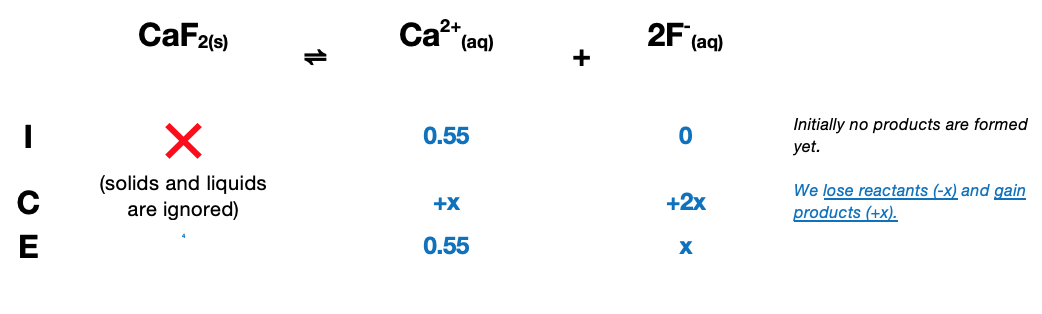# Problem: Calculate the molar solubility of CaF2 in a solution containing 0.550 M of Ca(NO3)2. The Ksp value for CaF2 is 1.46 x 10–10.

###### FREE Expert Solution

CaF2(s) will break up into its ions:              CaF2(s)  Ca2+(aq) + 2 F-(aq)

Ca(NO­3)2(s) also break up to its ions:       Ca(NO­3)2(s)  Ca2+(aq) + 2 NO3-(aq)

There is now an initial concentration of Ca2+ from Ca(NO­3)2(s):  this is called the Common Ion Effect

[Ca(NO3)2] = [Ca2+] = 0.55 M

Set up an ICE Table: CaF2(s)  Ca2+(aq) + 2 F-(aq)$\overline{){{\mathbf{K}}}_{{\mathbf{sp}}}{\mathbf{=}}\frac{\mathbf{products}}{\overline{)\mathbf{reactants}}}{\mathbf{=}}\mathbf{\left[}{\mathbf{Ca}}^{\mathbf{2}\mathbf{+}}\mathbf{\right]}{\mathbf{\left[}{\mathbf{F}}^{\mathbf{-}}\mathbf{\right]}}^{{\mathbf{2}}}}$

Substitute equilibrium concentrations and solve for x:

83% (481 ratings)###### Problem Details

Calculate the molar solubility of CaF2 in a solution containing 0.550 M of Ca(NO3)2. The Ksp value for CaF2 is 1.46 x 10–10.

Frequently Asked Questions

What scientific concept do you need to know in order to solve this problem?

Our tutors have indicated that to solve this problem you will need to apply the Ksp concept. You can view video lessons to learn Ksp. Or if you need more Ksp practice, you can also practice Ksp practice problems.

What professor is this problem relevant for?

Based on our data, we think this problem is relevant for Professor Wang's class at SPCOLLEGE.